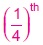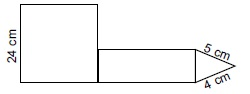# User Forum

Subject :IMO    Class : Class 5

The given figure (not drawn to scale) is made up of a square, a triangle and a rectangle. The breadth of the rectangle isthe length of the edge of the square. If the sum of the area of the square and the rectangle is 732 sq. cm, then find the perimeter of the figure.A112 cm
B118 cm
C210 cm
D151 cm

Class : Class 5
151

Class : Class 6
151 cm

Class : Class 5
210